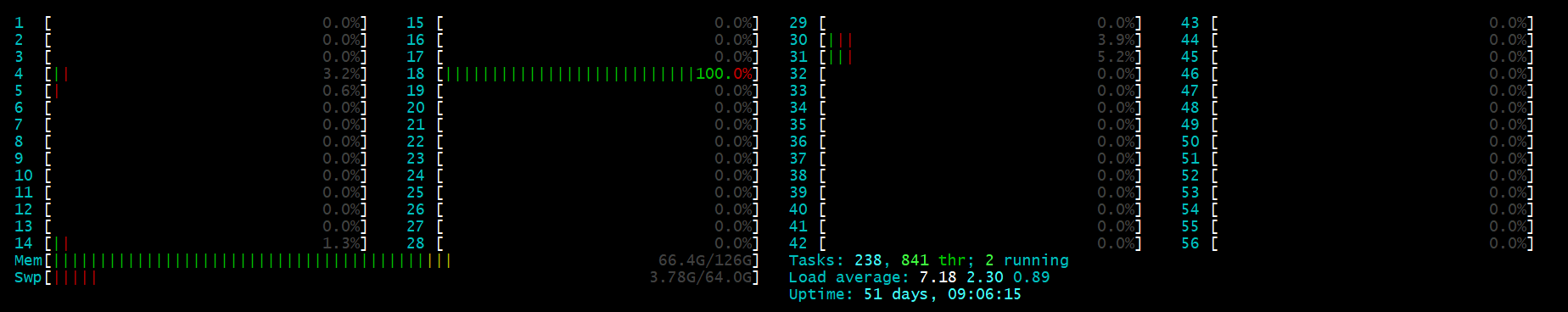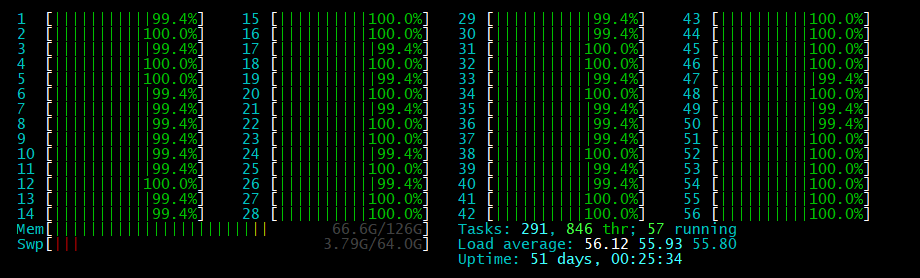# Python Multiprocessing 多进程，使用多核CPU计算 并使用tqdm显示进度条

1.背景

在python运行一些，计算复杂度比较高的函数时，服务器端单核CPU的情况比较耗时，因此需要多CPU使用多进程加快速度

2.函数要求

笔者使用的是：pathos.multiprocessing 库，进度条显示用tqdm库，安装方法：

pip install pathos


安装完成后

from pathos.multiprocessing import ProcessingPool as Pool
from tqdm import tqdm

　这边使用pathos的原因是因为，multiprocessing 库中的Pool 函数只支持单参数输入，例如 f(x) = x**2，而不能处理 f (x,y) = x+y 这类的函数　更不用说一些需要参数的函数 例如：F(x , alpha=0.5, gamma = 0.1) 这样。

3.代码

定义一个 函数 F [ X ] ,其中，输入X是可以在第一个维度上迭代的array, 大小：[ num_X, len ] , 在第一维度 num_X 上进行迭代。

def F(X,lamda=10,weight=0.05):

res={}

res.update(F_1(X,lamda=lamda,weight=weight))
res.update(F_2(X,lamda=lamda,weight=weight))
return res


x 是 F 的输出，是一个dict (字典格式)这里的两个函数超参数 lamda 和 weight 虽然每次调用的时候值是一样的，但是还是需要放一个数组每次用于迭代。
zip_lamda = [lamda for i in range(len(X)) ]
zip_weight = [weight for i in range(len(X)) ]

with tqdm(total=len(cold_sequences)) as t:
for i, x in enumerate(pool.imap(F,X,zip_lamda,zip_weight)):
X[i,:] = [x[key] for key in x.keys()]
Y[i,] = 0
t.update()

pool.close()
pool.join()


4.结果

mutiprocess 加速前mutiprocess 加速后posted @ 2019-07-26 04:59  SiyuanChen  阅读(4475)  评论(0编辑  收藏  举报